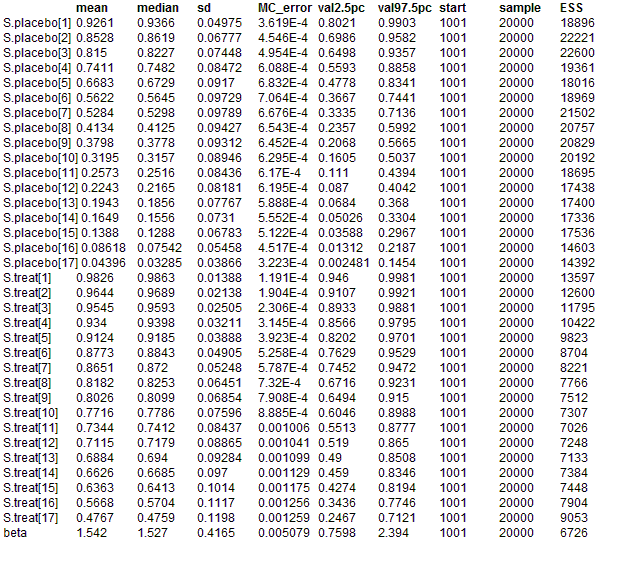#### Leuk: Cox regression

Several authors have discussed Bayesian inference for censored survival data where the integrated baseline hazard function is to be estimated non-parametrically Kalbfleisch (1978), Kalbfleisch and Prentice (1980), Clayton (1991), Clayton (1994). Clayton (1994) formulates the Cox model using the counting process notation introduced by Andersen and Gill (1982) and discusses estimation of the baseline hazard and regression parameters using MCMC methods. Although his approach may appear somewhat contrived, it forms the basis for extensions to random effect (frailty) models, time-dependent covariates, smoothed hazards, multiple events and so on. We show below how to implement this formulation of the Cox model in BUGS.

For subjects i = 1,...,n, we observe processes Ni(t) which count the number of failures which have occurred up to time t. The corresponding intensity process Ii(t) is given by

Ii(t)dt = E(dNi(t) | Ft-)

where dNi(t) is the increment of Ni over the small time interval [t, t+dt), and Ft- represents the available data just before time t. If subject i is observed to fail during this time interval, dNi(t) will take the value 1; otherwise dNi(t) = 0. Hence E(dNi(t) | Ft-) corresponds to the probability of subject i failing in the interval [t, t+dt). As dt -> 0 (assuming time to be continuous) then this probability becomes the instantaneous hazard at time t for subject i. This is assumed to have the proportional hazards form

Ii(t) = Yi(t)λ0(t)exp(βzi)

where Yi(t) is an observed process taking the value 1 or 0 according to whether or not subject i is observed at time t and λ0(t)exp(βzi) is the familiar Cox regression model. Thus we have observed data D = Ni(t), Yi(t), zi; i = 1,..n and unknown parameters β and Λ0(t) = Integral(λ0(u), u, t, 0), the latter to be estimated non-parametrically.    n

The joint posterior distribution for the above model is defined by

P(β, Λ0() | D) ~ P(D | β, Λ0()) P(β) P(Λ0())

For BUGS, we need to specify the form of the likelihood P(D | β, Λ0()) and prior distributions for β and Λ0(). Under non-informative censoring, the likelihood of the data is proportional to

Πi=1,...,n[Πt>=0Ιi(t)dNi(t)] exp(- Ii(t)dt)

This is essentially as if the counting process increments dNi(t) in the time interval [t, t+dt) are independent Poisson random variables with means Ii(t)dt:

dNi(t) ~ Poisson(Ii(t)dt)

We may write

Ii(t)dt = Yi(t)exp(βzi)dΛ0(t)

where dΛ0(t) = Λ0(t)dt is the increment or jump in the integrated baseline hazard function occurring during the time interval [t, t+dt). Since the conjugate prior for the Poisson mean is the gamma distribution, it would be convenient if Λ0() were a process in which the increments dΛ0(t) are distributed according to gamma distributions. We assume the conjugate independent increments prior suggested by Kalbfleisch (1978), namely

dΛ0(t) ~ Gamma(cdΛ*0(t), c)

Here, dΛ*0(t) can be thought of as a prior guess at the unknown hazard function, with c representing the degree of confidence in this guess. Small values of c correspond to weak prior beliefs. In the example below, we set dΛ*0(t) = r dt where r is a guess at the failure rate per unit time, and dt is the size of the time interval.

The above formulation is appropriate when genuine prior information exists concerning the underlying hazard function. Alternatively, if we wish to reproduce a Cox analysis but with, say, additional hierarchical structure, we may use the multinomial-Poisson trick described in the BUGS manual. This is equivalent to assuming independent increments in the cumulative `non-informative' priors. This formulation is also shown below.

The fixed effect regression coefficients β are assigned a vague prior

β ~ Normal(0.0, 0.000001)

BUGS language for the Leuk example:
model
{
# Set up data
for(i in 1:N) {
for(j in 1:T) {
# risk set = 1 if obs.t >= t
Y[i,j] <- step(obs.t[i] - t[j] + eps)
# counting process jump = 1 if obs.t in [ t[j], t[j+1] )
# i.e. if t[j] <= obs.t < t[j+1]
dN[i, j] <- Y[i, j] * step(t[j + 1] - obs.t[i] - eps) * fail[i]
}
}
# Model
for(j in 1:T) {
for(i in 1:N) {
dN[i, j] ~ dpois(Idt[i, j]) # Likelihood
Idt[i, j] <- Y[i, j] * exp(beta * Z[i]) * dL0[j]    # Intensity
}
dL0[j] ~ dgamma(mu[j], c)
mu[j] <- dL0.star[j] * c # prior mean hazard

# Survivor function = exp(-Integral{l0(u)du})^exp(beta*z)
S.treat[j] <- pow(exp(-sum(dL0[1 : j])), exp(beta * -0.5));
S.placebo[j] <- pow(exp(-sum(dL0[1 : j])), exp(beta * 0.5));
}
c <- 0.001
r <- 0.1
for (j in 1 : T) {
dL0.star[j] <- r * (t[j + 1] - t[j])
}
beta ~ dnorm(0.0,0.000001)
}

##### Data
``` list(N = 42, T = 17, eps = 1.0E-10,      obs.t = c(1, 1, 2, 2, 3, 4, 4, 5, 5, 8, 8, 8, 8, 11, 11, 12, 12, 15, 17, 22, 23, 6,       6, 6, 6, 7, 9, 10, 10, 11, 13, 16, 17, 19, 20, 22, 23, 25, 32, 32, 34, 35),      fail = c(1, 1, 1, 1, 1, 1, 1, 1, 1, 1, 1, 1, 1, 1, 1, 1, 1, 1, 1, 1, 1, 1, 1, 1, 0, 1, 0, 1, 0, 0, 1, 1, 0, 0, 0, 1, 1, 0, 0, 0, 0, 0),      Z = c(0.5, 0.5, 0.5, 0.5, 0.5, 0.5, 0.5, 0.5, 0.5, 0.5, 0.5, 0.5, 0.5, 0.5, 0.5, 0.5, 0.5, 0.5, 0.5, 0.5, 0.5,         -0.5, -0.5, -0.5, -0.5, -0.5, -0.5, -0.5, -0.5, -0.5, -0.5, -0.5, -0.5, -0.5, -0.5, -0.5, -0.5, -0.5, -0.5, -0.5, -0.5, -0.5),      t = c(1, 2, 3, 4, 5, 6, 7, 8, 10, 11, 12, 13, 15, 16, 17, 22, 23, 35)) ```
##### Inits for chain 1
``` list( beta = 0.0, dL0 = c(1.0,1.0,1.0,1.0,1.0,1.0,1.0,1.0,1.0,1.0,1.0,1.0,1.0,1.0,1.0, 1.0,1.0)) ```

##### Inits for chain 2
``` list( beta = 1.0, dL0 = c(2.0,2.0,2.0,2.0,2.0,2.0,2.0,2.0,2.0,2.0,2.0,2.0,2.0,2.0,2.0, 2.0,2.0)) ```
Results